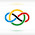### 2018 European Mathematical Cup Junior #2

Find all pairs $(x; y)$ of positive integers such that
$$xy | x^2 + 2y -1.$$

We have $x^2 + 2y - 1\equiv 0 \pmod x\implies 2y - 1\equiv 0 \pmod x$ or $2y - 1 = xz$ for some positive integer $z$. Now we have $y\mid x+z$ or$\frac{xz+1}{2}\mid x+z\implies xz+1\le 2(x+z) \implies (x-2)(z-2)\le 3.$For $x = 1$, $\frac{z+1}{2}\mid z+1$ which is true for any odd natural $z$. For $x = 3$, we have $z \le 5$ which on checking gives $z = 1$ or $z = 5$. For $x = 5$, $z = 3$, and for $z=1$, $x$ is any odd. So we have solutions:$(x,y) = (1,k),(3,2),(3,8),(5,8),(2k-1,k) ~~\text{for any positive integer } k.$

However, note that the $(3,2)$ case is the same as $(2k-1, k)$, so our only solutions are $\boxed{(x,y) = (1,k),(3,8),(5,8),(2k-1,k) ~~\text{for any positive integer } k.}$

$\square$

1.Anonymous12/31/2021

(3,2) is a special case of (2k-1,k)

2.@Anonymous

Thanks for pointing out the mistake!
I have fixed it now.